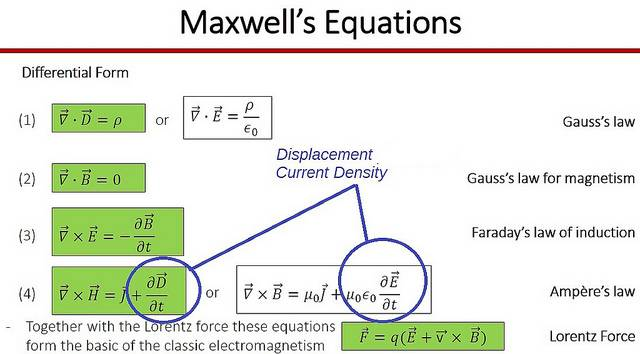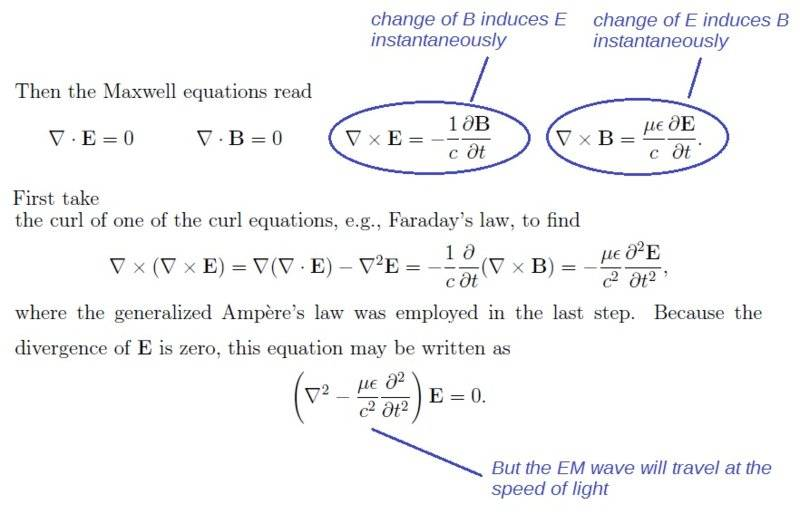# Maxwell's Law of Induction - How Does it Work?

ItFeelsGoodToBeAlive
So, I was studying Maxwell's equations and I don't really understand the last one - Ampere's Law (with Maxwell's extra term added in). The bit I'm not able to understand is the term Maxwell added. How exactly does a changing electric field through a closed loop induce a magnetic field along that loop?

I mean, Faraday's law of induction is a result of special relativity...what is Maxwell's law of induction a result of? (ie. how can whatever it is that's going on to cause the whole induction thing be explained physically?)in

Gold Member
The way Maxwell initially understood it was that he envisioned the electric field to be a dielectric polarization of space itself. In terms of virtual particles in QFT this still is not a bad way of look at it. So let's consider a physical version of this.

Imagine a crystal made of dipolar molecules. The net charge is zero but by compressing the crystal you caused the positive poles to move a bit to the right and the negative poles to move a bit to the left. There would then be a net charge current and that would momentarily induce a magnetic field. The polarization of the crystal is added to the vacuum E field to give the D field in the material. The E field itself can be viewed as this same sort of process happening at to the vacuum itself.

Note that this occurs dually with changing B fields. Even without magnetic charges we can set up a magnetic current by polarizing a diamagnetic medium. Say, set up a board with bar magnets on pivots. Have the N poles to the right and S poles to the left and then pivot them all together. You induce a net N current to the left or S to the right. This magnetic current will induce a corresponding E field. This applies to any changing B field, there's some form of effective magnetic charge current and it induces an E field. That's Faraday's law of induction in action and that's how the B field induces current in a loop. But note that it is enhanced in ferromagnetic media where dipole allignments will enhance the B field and you get a higher induced E field.

The Maxwell terms says that E field acts in a dual way. The only break in this symmetry is our absence of magnetic monopole charges (so far as we've ever been able to discover).

Gold Member
The bit I'm not able to understand is the term Maxwell added. How exactly does a changing electric field through a closed loop induce a magnetic field along that loop?
I thought that the electric field acted around the loop and cause a magnetic field going through the loop.

alan123hk
So, I was studying Maxwell's equations and I don't really understand the last one - Ampere's Law (with Maxwell's extra term added in). The bit I'm not able to understand is the term Maxwell added. How exactly does a changing electric field through a closed loop induce a magnetic field along that loop?
It is because change in electric flux density (also known as displacement current density) creates magnetic field in the same way as the Faraday's law. For example, the change of electric flux density between two plates of a capacitor will induce circular magnetic field around the space between the two plates as though a current were present there as well.

I mean, Faraday's law of induction is a result of special relativity...what is Maxwell's law of induction a result of?

According to relativity, magnetic and electric fields are really not different fields, what is seen as a magnetic field by one observer could be seen as an electric field by another observer and vice versa, since all such observers are equal, no one has more right than another, therefore, I don't see the reason why the change of electric field not inducing magnetic field as well.

When an electric charge is wiggle back-and-forth, a wiggling electric field is created, which, in turn, creates a wiggling magnetic field, which creates a wiggling electric field, and gain and again in the same way, these wiggles are in fact spreading out of free electromagnetic waves (radio waves).
View attachment 236877

Last edited:
Gold Member
When an electric charge is wiggle back-and-forth, a wiggling electric field is created, which, in turn, creates a wiggling magnetic field, which creates a wiggling electric field, and gain and again in the same way, these wiggles are in fact spreading out of free electromagnetic waves (radio waves).

https://www.physicsforums.com/attachments/236863
But for an EM wave the electric and magnetic fields are in-phase. The two fields are not exchanging energy but each have half the energy?

alan123hk
But for an EM wave the electric and magnetic fields are in-phase. The two fields are not exchanging energy but each have half the energy?

Yes, the EM wave is propagated with the electric and magnetic field vectors in phase and perpendicular, they interact with each other in a close and instant way.

I agree that the way of saying "electric field is created, which, in turn, creates a wiggling magnetic field... and again and again in the same way... are electromagnetic waves" may seems somewhat inappropriate, there is a risk of misleading people to think that the electric and magnetic field are separated in some way as the EM wave propagates, although I have actually seen the same explanation of EM wave in a science book many years ago.#### Attachments

Last edited:
Gold Member
2022 Award
It is very misleading to say that an "electric field is created, which, in turn, creates a wiggling magnetic field... and again and again in the same way... are electromagnetic waves". To begin with, there's only one electromagnetic field, represented in the classical form by its electric and magnetic components ##\vec{E}## and ##\vec{B}## (I refer to the microscopic fundamental laws; the further split into free and internal fields in macroscopic electromagnetics is another more complicated topic of its own).

The Maxwell equations split into two classes, the homogeneous equations
$$\vec{\nabla} \times \vec{E}+\partial_t \vec{B}=0, \quad \vec{\nabla} \cdot \vec{B}=0,$$
and the inhomogeneous ones
$$\vec{\nabla} \times \vec{B} - \frac{1}{c^2} \partial_t \vec{E}=\mu_0 \vec{j}, \quad \vec{\nabla} \cdot \vec{E}=\frac{1}{\epsilon_0} \rho.$$
The homogeneous equations are constraint equations for the field components, and the inhomogeneous equations provide ##\rho## and ##\vec{j}## as the sources. This becomes clear from the fact that the solutions of the equations are the retarded fields given in terms of the retarded propagator with the charge-current distributions as the causal (!) sources. See, e.g.,

https://en.wikipedia.org/wiki/Jefimenko's_equations

particularly the section "Discussion" therein.

alan123hk
The homogeneous equations are constraint equations for the field components, and the inhomogeneous equations provide ρρ\rho and →jj→\vec{j} as the sources. This becomes clear from the fact that the solutions of the equations are the retarded fields given in terms of the retarded propagator with the charge-current distributions as the causal (!) sources. See, e.g.,

Charge-current distributions is certainly the source of EM field no matter it is static or varying. However, the electromagnetic waves can still propagate in the space even after the source ceased to exist, so the problem is whether or not we can explain the propagation mechanism of EM wave in words as looping of mutual induction of electric and magnetic fields.